## Go Back N Protocol-

Before you go through this article, make sure that you have gone through the previous article on Go back N Protocol.

We have discussed-

• Sliding window protocols allow the sender to send multiple frames before needing acknowledgements.
• Go back N is an implementation of a sliding window protocol.

In this article, we will discuss practice problems based on Go back N protocol.

## Problem-01:

A 20 Kbps satellite link has a propagation delay of 400 ms. The transmitter employs the “go back n ARQ” scheme with n set to 10.

Assuming that each frame is 100 bytes long, what is the maximum data rate possible?

1. 5 Kbps
2. 10 Kbps
3. 15 Kbps
4. 20 Kbps

## Solution-

Given-

• Bandwidth = 20 Kbps
• Propagation delay (Tp) = 400 ms
• Frame size = 100 bytes
• Go back N is used where N = 10

### Calculating Transmission Delay-

Transmission delay (Tt)

= Frame size / Bandwidth

= 100 bytes / 20 Kbps

= (100 x 8 bits) / (20 x 10bits per sec)

= 0.04 sec

= 40 msec

### Calculating Value Of ‘a’-

a = Tp / Tt

a = 400 msec / 40 msec

a = 10

### Calculating Efficiency-

Efficiency (η)

= N / (1+2a)

= 10 / (1 + 2 x 10)

= 10 / 21

= 0.476

= 47.6 %

### Calculating Maximum Data Rate Possible-

Maximum data rate possible or Throughput

= Efficiency x Bandwidth

= 0.476 x 20 Kbps

= 9.52 Kbps

≅ 10 Kbps

Thus, Correct Option is (B)

## Problem-02:

Consider the Go back N protocol with a sender’s window size of ‘n’. Suppose that at time ‘t’, the next inorder packet the receiver is expecting has a sequence number of ‘K’. Assume that the medium does not reorder messages.

Answer the following questions-

### Part-01:

What are the possible sets of sequence numbers inside the sender’s window at time ‘t’. Assume the sender has already received the ACKs.

1. [K-1, K+n-1]
2. [K, K+n-1]
3. [K, K+n]
4. [K+n, K-1]

### Part-02:

If acknowledgements are still on their way to sender, what are all possible values of the ACK field in the messages currently propagating back to the sender at a time ‘t’?

1. [K-n, K-1]
2. [K-1, K-n]
3. [K, K-n]
4. [K-n, K+1]

## Solution-

### Part-01:

• In Go back N protocol, the receiver window size is 1.
• It is given that receiver expects the packet having sequence number ‘K’.
• It means it has processed all the packets ranging from 0 to K-1.
• It is given that sender has received the acknowledgement for all these packets.
• So, outstanding packets in sender’s window waiting for the acknowledgement starts from K.
• Sender window size = n.
• Therefore, last packet in sender’s window will have sequence number K+n-1.

Thus, Option (B) is correct.

### Part-02:

• Acknowledgement number is the next expected sequence number by the receiver.
• Receiver expects the packet having sequence number ‘K’ at time ‘t’.
• It means it has received the packets ranging from 0 to K-1 whose acknowledgements are are on the way.
• For the (K-1)th packet, acknowledgement number would be ‘K’.
• For the (K-2)th packet, acknowledgement number would be ‘K-1’ and so on.

Now,

• At any time, maximum number of outstanding packets can be ‘n’.
• This is because sender’s window size is ‘n’.
• Therefore, the possible values of acknowledgement number ranges from [K-n+1, ……, K-3, K-2, K-1, K] (total n values)
• Here, we have assumed that the acknowledgement for all the packets are sent independently.

Thus, Option (C) is correct.

## Problem-03:

Station A needs to send a message consisting of 9 packets to station B  using a sliding window (window size 3) and go back n error control strategy. All packets are ready and immediately available for transmission.

If every 5th packet that A transmits gets lost (but no ACKs from B ever get lost), then what is the number of packets that A will transmit for sending the message to B?

1. 12
2. 14
3. 16
4. 18

## Solution-

Given-

• Total number of packets to be sent = 9
• Go back N is used where N = 3
• Every 5th packet gets lost

### Step-01:

Since sender window size is 3, so sender sends 3 packets (1, 2, 3)-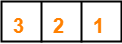Total packets sent till now from sender side = 3

### Step-02:

After receiving the acknowledgement for packet-1, sender slides its window and sends packet-4.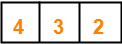Total packets sent till now from sender side = 4

### Step-03:

After receiving the acknowledgement for packet-2, sender slides its window and sends packet-5.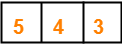Total packets sent till now from sender side = 5

### Step-04:

After receiving the acknowledgement for packet-3, sender slides its window and sends packet-6.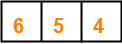Total packets sent till now from sender side = 6

### Step-05:

After receiving the acknowledgement for packet-4, sender slides its window and sends packet-7.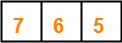Total packets sent till now from sender side = 7

### Step-06:

• According to question, every 5th packet gets lost.
• So, packet-5 gets lost and when time out occurs, sender retransmits packet-5.
• In Go back N, all the following packets are also discarded by the receiver.
• So, packet-6 and packet-7 are discarded by the receiver and they are also retransmitted.
• Thus, the entire window is retransmitted.

So, we have-Total packets sent till now from sender side = 10

Now, the next 5th packet that will be lost will be packet-7. (6, 7, 5, 6, 7)

### Step-07:

After receiving the acknowledgement for packet-5, sender slides its window and sends packet-8.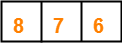Total packets sent till now from sender side = 11

### Step-08:

After receiving the acknowledgement for packet-6, sender slides its window and sends packet-9.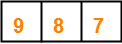Total packets sent till now from sender side = 12

### Step-09:

• According to question, every 5th packet gets lost.
• So, packet-7 gets lost and when time out occurs, sender retransmits packet-7 and the following packets.
• Thus, the entire window is retransmitted.

So, we have-Total packets sent till now from sender side = 15

Now, the next 5th packet that will be lost will be packet-9. (8, 9, 7, 8, 9)

### Step-10:

After receiving the acknowledgement for packet-7, sender slides its window.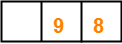Total packets sent till now from sender side = 15

### Step-11:

After receiving the acknowledgement for packet-8, sender slides its window.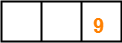Total packets sent till now from sender side = 15

### Step-12:

• According to question, every 5th packet gets lost.
• So, packet-9 gets lost and when time out occurs, sender retransmits packet-9.

So, we have-Total packets sent till now from sender side = 16

Finally, all the 9 packets got transmitted which took total 16 number of transmissions.

Thus, Correct Option is (C).

## Problem-04:

In Go back 4, if every 6th packet that is being transmitted is lost and if total number of packets to be sent is 10, then how many transmissions will be required?

## Solution-

• Try yourself!
• We have to solve in exactly the same way as we have solved Problem-03.
• Total number of transmissions required will be 17.

## Problem-05:

A 1 Mbps satellite link connects two ground stations. The altitude of the satellite is 36504 km and speed of the signal is 3 x 108 m/sec. What should be the packet size for a channel utilization of 25% for a satellite link using go back 127 sliding window protocol?

1. 120 bytes
2. 60 bytes
3. 240 bytes
4. 90 bytes

## Solution-

Given-

• Bandwidth = 1 Mbps
• Distance = 2 x 36504 km = 73008 km
• Propagation speed = 3 x 108 m/sec
• Efficiency = 25% = 1/4
• Go back N is used where N = 127

Let the packet size be L bits.

### Calculating Transmission Delay-

Transmission delay (Tt)

= Packet size / Bandwidth

= L bits / 1 Mbps

= L μsec

### Calculating Propagation Delay-

Propagation delay (Tp)

= Distance / Speed

= (73008 x 103 m) / (3 x 108 m/sec)

= 24336 x 10-5 sec

= 243360 μsec

### Calculating Value of ‘a’-

a = Tp / Tt

a = 243360 μsec / L μsec

a = 243360 / L

### Calculating Packet Size-

Efficiency (η) = N / (1+2a)

Substituting the values, we get-

1/4 = 127 / (1 + 2 x 243360 / L)

1/4 = 127 x L / (L + 486720)

L + 486720 = 508 x L

507 x L = 486720

L = 960

From here, packet size = 960 bits or 120 bytes.

Thus, Correct Option is (A).

## Problem-06:

Consider a network connecting two systems located 8000 km apart. The bandwidth of the network is 500 x 106 bits per second. The propagation speed of the media is 4 x 106 meters per second. It is needed to design a Go back N sliding window protocol for this network. The average packet size is 107 bits. The network is to be used to its full capacity.

Assume that processing delays at nodes are negligible. Then, the minimum size in bits of the sequence number field has to be ______ ?

## Solution-

Given-

• Distance = 8000 km
• Bandwidth = 500 x 106 bps
• Propagation speed = 4 x 106 m/sec
• Packet size = 107 bits

Now,

• For using the network to its full capacity, Efficiency (η) = 1
• Efficiency (η) = 1 when sender window size = 1+2a

### Calculating Transmission Delay-

Transmission delay (Tt)

= Packet size / Bandwidth

= 107 bits / (500 x 106 bits per sec)

= 1 / 50 sec

= 0.02 sec

### Calculating Propagation Delay-

Propagation delay (Tp)

= Distance / Speed

= 8000 km / (4 x 106 m/sec)

= 2 sec

### Calculating Value of ‘a’-

a = Tp / Tt

a = 2 sec / 0.02 sec

a = 100

### Calculating Sender Window Size-

Sender window size

= 1 + 2a

= 1 + 2 x 100

= 201

### Calculating Minimum Size Of Sequence Number Field-

Minimum number of bits required in the sequence number field

= ⌈log2(1+2a)⌉

= ⌈log2(201)⌉

= ⌈7.65⌉

= 8

Thus, Minimum size of sequence number field = 8 bits.

Next Article- Selective Repeat Protocol

Get more notes and other study material of Computer Networks.

Watch video lectures by visiting our YouTube channel LearnVidFun.

## Sliding Window Protocol-

Before you go through this article, make sure that you have gone through the previous article on Sliding Window Protocol.

The two well known implementations of sliding window protocol are-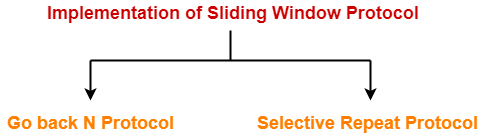1. Go back N Protocol
2. Selective Repeat Protocol

In this article, we will discuss about Go back N protocol.

## Go back N Protocol-

Go back N protocol is an implementation of a sliding window protocol.

The features and working of this protocol are explained in the following points-

## Point-01:

 In Go back N, sender window size is N and receiver window size is always 1.

In Go back N,

• Sender window size = N. Example in Go back 10, sender window size will be 10.
• Receiver window size is always 1 for any value of N.

## Point-02:

 Go back N uses cumulative acknowledgements.

In Go back N,

• Receiver maintains an acknowledgement timer.
• Each time the receiver receives a new frame, it starts a new acknowledgement timer.
• After the timer expires, receiver sends the cumulative acknowledgement for all the frames that are unacknowledged at that moment.

### NOTE-

• A new acknowledgement timer does not start after the expiry of old acknowledgement timer.
• It starts after a new frame is received.

## Point-03:

 Go back N may use independent acknowledgements too.

• The above point does not mean that Go back N can not use independent acknowledgements.
• Go back N may use independent acknowledgements too if required.
• The kind of acknowledgement used depends on the expiry of acknowledgement timer.

### Example-

• Consider after the expiry of acknowledgement timer, there is only one frame left to be acknowledged.
• Then, Go back N sends the independent acknowledgement for that frame.

## Point-04:

 Go back N does not accept the corrupted frames and silently discards them.

In Go back N,

• If receiver receives a frame that is corrupted, then it silently discards that frame.
• The correct frame is retransmitted by the sender after the time out timer expires.
• Silently discarding a frame means-

“Simply rejecting the frame and not taking any action”

(like not sending a NACK to the sender to send the correct frame)

## Point-05:

 Go back N does not accept out of order frames and silently discards them.

In Go back N,

• If receiver receives a frame whose sequence number is not what the receiver expects, then it silently discards that frame.
• All the following frames are also discarded.
• This is because receiver window size is 1 and therefore receiver can not accept out of order frames.

## Point-06:

 Go back N leads to retransmission of entire window if for any frame, no ACK is received by the sender.

In Go back N,

• Receiver silently discards the frame if it founds the frame to be either corrupted or out of order.
• It does not send any acknowledgement for such frame.
• It silently discards the following frames too.

Thus,

• If for any particular frame, sender does not receive any acknowledgement, then it understands that along with that frame, all the following frames must also have been discarded by the receiver.
• So, sender has to retransmit all the following frames too along with that particular frame.
• Thus, it leads to the retransmission of entire window.
• That is why, the protocol has been named as “Go back N“.

## Point-07:

 Go back N leads to retransmission of lost frames after expiry of time out timer.

In Go back N,

• Consider a frame being sent to the receiver is lost on the way.
• Then, it is retransmitted only after time out timer expires for that frame at sender’s side.

## Efficiency of Go back N-

Efficiency of any flow control protocol is given by-

 Efficiency = Sender Window Size in Protocol / (1 + 2a)

In Go back N protocol, sender window size = N.

Thus,

 Efficiency of Go back N = N / (1 + 2a)

To gain better understanding about Go back N ARQ,

Watch this Video Lecture

Next Article- Practice Problems On Go Back N Protocol

Get more notes and other study material of Computer Networks.

Watch video lectures by visiting our YouTube channel LearnVidFun.

## Flow Control Protocols-

In computer networking, there are various flow control protocols-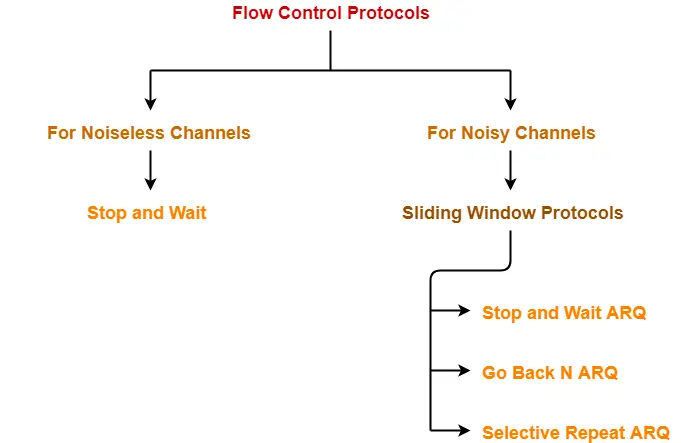In this article, we will discuss about sliding window protocol.

## Sliding Window Protocol-

• Sliding window protocol is a flow control protocol.
• It allows the sender to send multiple frames before needing the acknowledgements.
• Sender slides its window on receiving the acknowledgements for the sent frames.
• This allows the sender to send more frames.
• It is called so because it involves sliding of sender’s window.

 Maximum number of frames that sender can send without acknowledgement= Sender window size

## Optimal Window Size-

 In a sliding window protocol, optimal sender window size = 1 + 2a

### Derivation-

We know,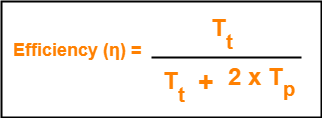To get 100% efficiency, we must have-

η = 1

Tt / (Tt + 2Tp) = 1

Tt = Tt + 2Tp

Thus,

• To get 100% efficiency, transmission time must be Tt + 2Tinstead of Tt.
• This means sender must send the frames in waiting time too.
• Now, let us find the maximum number of frames that can be sent in time Tt + 2Tp.

We have-

• In time Tt, sender sends one frame.
• Thus, In time Tt + 2Tp, sender can send (Tt + 2Tp) / Tt frames i.e. 1+2a frames.

Thus, to achieve 100% efficiency, window size of the sender must be 1+2a.

## Required Sequence Numbers-

• Each sending frame has to be given a unique sequence number.
• Maximum number of frames that can be sent in a window = 1+2a.
• So, minimum number of sequence numbers required = 1+2a.

 To have 1+2a sequence numbers,Minimum number of bits required in sequence number field = ⌈log2(1+2a)⌉

## NOTE-

• When minimum number of bits is asked, we take the ceil.
• When maximum number of bits is asked, we take the floor.

## Choosing a Window Size-

The size of the sender’s window is bounded by-

### 1. Receiver’s Ability-

• Receiver’s ability to process the data bounds the sender window size.
• If receiver can not process the data fast, sender has to slow down and not transmit the frames too fast.

### 2. Sequence Number Field-

• Number of bits available in the sequence number field also bounds the sender window size.
• If sequence number field contains n bits, then 2n sequence numbers are possible.
• Thus, maximum number of frames that can be sent in one window = 2n.

 For n bits in sequence number field, Sender Window Size = min (1+2a , 2n)

## Implementations of Sliding Window Protocol-

The two well known implementations of sliding window protocol are-## Efficiency-

Efficiency of any flow control protocol may be expressed as-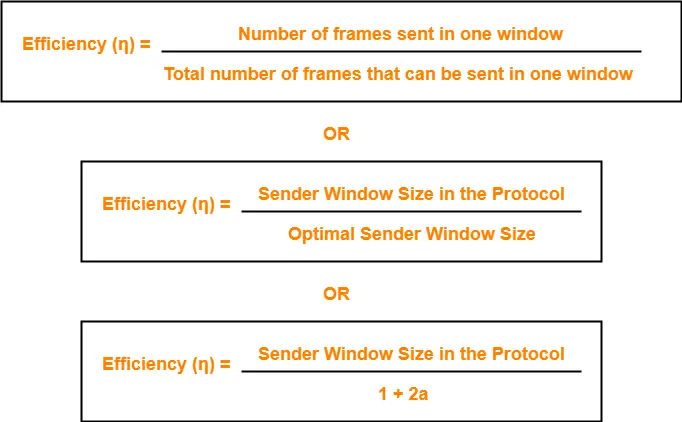### Example-

In Stop and Wait ARQ, sender window size = 1.

Thus,

Efficiency of Stop and Wait ARQ = 1 / 1+2a

## Problem-01:

If transmission delay and propagation delay in a sliding window protocol are 1 msec and 49.5 msec respectively, then-

1. What should be the sender window size to get the maximum efficiency?
2. What is the minimum number of bits required in the sequence number field?
3. If only 6 bits are reserved for sequence numbers, then what will be the efficiency?

## Solution-

Given-

• Transmission delay = 1 msec
• Propagation delay = 49.5 msec

### Part-01:

To get the maximum efficiency, sender window size

= 1 + 2a

= 1 + 2 x (Tp / Tt)

= 1 + 2 x (49.5 msec / 1 msec)

= 1 + 2 x 49.5

= 100

Thus,

For maximum efficiency, sender window size = 100

### Part-02:

Minimum number of bits required in the sequence number field

= ⌈log2(1+2a)⌉

= ⌈log2(100)⌉

= ⌈6.8⌉

= 7

Thus,

Minimum number of bits required in the sequence number field = 7

### Part-03:

If only 6 bits are reserved in the sequence number field, then-

Maximum sequence numbers possible = 26 = 64

Now,

Efficiency

= Sender window size in the protocol / Optimal sender window size

= 64 / 100

= 0.64

= 64%

## Problem-02:

If transmission delay and propagation delay in a sliding window protocol are 1 msec and 99.5 msec respectively, then-

1. What should be the sender window size to get the maximum efficiency?
2. What is the minimum number of bits required in the sequence number field?
3. If only 7 bits are reserved for sequence numbers, then what will be the efficiency?

## Solution-

Given-

• Transmission delay = 1 msec
• Propagation delay = 99.5 msec

### Part-01:

To get the maximum efficiency, sender window size

= 1 + 2a

= 1 + 2 x (Tp / Tt)

= 1 + 2 x (99.5 msec / 1 msec)

= 1 + 2 x 99.5

= 200

Thus,

For maximum efficiency, sender window size = 200

### Part-02:

Minimum number of bits required in the sequence number field

= ⌈log2(1+2a)⌉

= ⌈log2(200)⌉

= ⌈7.64⌉

= 8

Thus,

Minimum number of bits required in the sequence number field = 8

### Part-03:

If only 6 bits are reserved in the sequence number field, then-

Maximum sequence numbers possible = 27 = 128

Now,

Efficiency

= Sender window size in the protocol / Optimal sender window size

= 128 / 200

= 0.64

= 64%

To gain better understanding about sliding window protocol,

Watch this Video Lecture

Next Article- Practice Problems On Sliding Window Protocol

Get more notes and other study material of Computer Networks.

Watch video lectures by visiting our YouTube channel LearnVidFun.

## Stop and Wait Protocol-

Before you go through this article, make sure that you have gone through the previous article on Stop and Wait Protocol.

We have discussed-

• Stop and wait protocol is a simplest flow control protocol.
• Sender sends a data packet to the receiver and then waits for its acknowledgement.
• On receiving the acknowledgement, sender sends the next data packet.

Also Read- Stop and Wait ARQ

In this article, we will discuss practice problems based on stop and wait protocol.

## Problem-01:

If the bandwidth of the line is 1.5 Mbps, RTT is 45 msec and packet size is 1 KB, then find the link utilization in stop and wait.

## Solution-

Given-

• Bandwidth = 1.5 Mbps
• RTT = 45 msec
• Packet size = 1 KB

### Calculating Transmission Delay-

Transmission delay (Tt)

= Packet size / Bandwidth

= 1 KB / 1.5 Mbps

= (210 x 8 bits) / (1.5 x 106 bits per sec)

= 5.461 msec

### Calculating Propagation Delay-

Propagation delay (Tp)

= Round Trip Time / 2

= 45 msec / 2

= 22.5 msec

### Calculating Value Of ‘a’-

a = Tp / Tt

a = 22.5 msec / 5.461 msec

a = 4.12

### Calculating Link Utilization-

Link Utilization or Efficiency (η)

= 1 / 1+2a

= 1 / (1 + 2 x 4.12)

= 1 / 9.24

= 0.108

= 10.8 %

## Problem-02:

A channel has a bit rate of 4 Kbps and one way propagation delay of 20 msec. The channel uses stop and wait protocol. The transmission time of the acknowledgement frame is negligible. To get a channel efficiency of at least 50%, the minimum frame size should be-

1. 80 bytes
2. 80 bits
3. 160 bytes
4. 160 bits

## Solution-

Given-

• Bandwidth = 4 Kbps
• Propagation delay (Tp) = 20 msec
• Efficiency >= 50%

Let the required frame size = L bits.

### Calculating Transmission Delay-

Transmission delay (Tt)

= Packet size / Bandwidth

= L bits / 4 Kbps

### Calculating Value Of ‘a’-

a = Tp / Tt

a = 20 msec / ( L bits / 4 Kbps)

a = (20 msec x 4 Kbps) / L bits

### Condition For Efficiency To Be At least 50%-

For efficiency to be at least 50%, we must have-

1 / 1+2a >= 1/2

a <= 1/2

Substituting the value of ‘a’, we get-

(20 msec x 4 Kbps) / L bits <= 1/2

L bits >= (20 msec x 4 Kbps) x 2

L bits >= (20 x 10-3 sec x 4 x 103 bits per sec) x 2

L bits >= 20 x 4 bits x 2

L >= 160

From here, frame size must be at least 160 bits.

Thus, Correct Option is (D).

## Problem-03:

What is the throughput achievable in stop and wait protocol by a maximum packet size of 1000 bytes and network span of 10 km.

Assume the speed of light in cable is 70% of the speed of light in vaccum.

## Solution-

We have-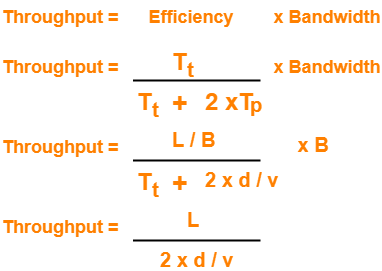• In the given question, we are not provided with the network’s bandwidth.
• So, in the above formula of throughput, we have ignored the term Tt from the denominator.
• Although it is incorrect, but we still ignore it for solving the question.

Now, Given-

• L = 1000 bytes
• d = 10 km = 104 m
• v = 70% of 3 x 108 m/sec = 2.1 x 108 m/sec

Substituting the values in the above relation, we get-

Throughput

= 1000 bytes / [ 2 x 104 m / (2.1 x 108 m/sec)]

= 1.05 x 107 bytes per sec

= 10.5 MBps

## Problem-04:

If the packet size is 1 KB and propagation time is 15 msec, the channel capacity is 109 b/sec, then find the transmission time and utilization of sender in stop and wait protocol.

## Solution-

Given-

• Packet size = 1 KB
• Propagation time (Tp) = 15 msec
• Channel capacity = Bandwidth (here) = 109 b/sec

### NOTE-

• Generally, channel capacity is the total number of bits which a channel can hold. So, its unit is bits.
• But here, channel capacity is actually given as bandwidth because its unit is b/sec.

### Calculating Transmission Delay-

Transmission delay (Tt)

= Packet size / Bandwidth

= 1 KB / 109 bits per sec

= 210 bits / 109 bits per sec

= 1.024 μsec

### Calculating Value Of ‘a’-

a = Tp / Tt

a = 15 msec / 1.024 μsec

a = 15000 μsec / 1.024 μsec

a = 14648.46

### Calculating Sender Utilization-

Sender Utilization or Efficiency (η)

= 1 / 1+2a

= 1 / (1 + 2 x 1468.46)

= 1 / 29297.92

= 0.0000341

= 0.00341 %

## Problem-05:

Consider a MAN with average source and destination 20 Km apart and one way delay of 100 μsec. At what data rate does the round trip delay equals the transmission delay for a 1 KB packet?

## Solution-

Given-

• Distance = 20 Km
• Propagation delay (Tp) = 100 μsec
• Packet size = 1 KB

We need to have-

Round Trip Time = Transmission delay

2 x Propagation delay = Transmission delay

Substituting the values in the above relation, we get-

2 x 100 μsec = 1 KB / Bandwidth

Bandwidth = 1 KB / 200 μsec

Bandwidth = (210 x 106 / 200 ) bytes per sec

Bandwidth = 5.12 MBps or 40.96 Mbps

## Problem-06:

Consider two hosts X and Y connected by a single direct link of rate 106 bits/sec. The distance between the two hosts is 10,000 km and the propagation speed along the link is 2 x 108 m/sec. Host X sends a file of 50,000 bytes as one large message to host Y continuously. Let the transmission and propagation delays be p milliseconds and q milliseconds respectively.

Then the value of p and q are-

1. p = 50 and q = 100
2. p = 50 and q = 400
3. p = 100 and q = 50
4. p = 400 and q = 50

## Solution-

Given-

• Bandwidth = 106 bits/sec
• Distance = 10,000 km
• Propagation speed = 2 x 108 m/sec
• Packet size = 50,000 bytes

### Calculating Transmission Delay-

Transmission delay (Tt)

= Packet size / Bandwidth

= 50000 bytes / 106 bits per sec

= (5 x 104 x 8 bits) / 106 bits per sec

= ( 4 x 105 bits ) / 106 bits per sec

= 0.4 sec

= 400 msec

### Calculating Propagation Delay-

Propagation delay (Tp)

= Distance / Propagation speed

= 10000 km / (2 x 108 m/sec)

= 107 m / (2 x 108 m/sec)

= 50 msec

Thus, Option (D) is correct.

## Problem-07:

The values of parameters for the stop and wait ARQ protocol are as given below-

• Bit rate of the transmission channel = 1 Mbps
• Propagation delay from sender to receiver = 0.75 ms
• Time to process a frame = 0.25 ms
• Number of bytes in the information frame = 1980
• Number of bytes in the acknowledge frame = 20
• Number of overhead bytes in the information frame = 20

Assume that there are no transmission errors. Then the transmission efficiency (in %) of the stop and wait ARQ protocol for the above parameters is ___________ . (correct to 2 decimal places)

## Solution-

Given-

• Bandwidth = 1 Mbps
• Propagation delay (Tp) = 0.75 ms
• Processing time (Tprocess) = 0.25 ms
• Data frame size = 1980 bytes
• Acknowledgement frame size = 20 bytes
• Overhead in data frame = 20 bytes

### Calculating Useful Time-

Useful data sent

= Transmission delay of useful data bytes sent

= Useful data bytes sent / Bandwidth

= (1980 bytes – 20 bytes) / 1 Mbps

= 1960 bytes / 1 Mbps

= (1960 x 8 bits) / (106 bits per sec)

= 15680 μsec

= 15.680 msec

### Calculating Total Time-

Total time

= Transmission delay of data frame + Propagation delay of data frame + Processing delay of data frame + Transmission delay of acknowledgement + Propagation delay of acknowledgement

= (1980 bytes / 1 Mbps) + 0.75 msec + 0.25 msec + (20 bytes / 1 Mbps) + 0.75 msec

= 15.840 msec + 0.75 msec + 0.25 msec + 0.160 msec + 0.75 msec

= 17.75 msec

### Calculating Efficiency-

Efficiency (η)

= Useful time / Total time

= 15.680 msec / 17.75 msec

= 0.8833

= 88.33%

## Problem-08:

A sender uses the stop and wait ARQ protocol for reliable transmission of frames. Frames are of size  1000 bytes and the transmission rate at the sender is 80 Kbps. Size of an acknowledgement is 100 bytes and the transmission rate at the receiver is 8 Kbps. The one way propagation delay is 100 msec.

Assuming no frame is lost, the sender throughput is __________ bytes/sec.

## Solution-

Given-

• Frame size = 1000 bytes
• Sender bandwidth = 80 Kbps
• Acknowledgement size = 100 bytes
• Receiver bandwidth = 8 Kbps
• Propagation delay (Tp) = 100 msec

### Calculating Transmission Delay Of Data Frame-

Transmission delay (Tt)

= Frame size / Sender bandwidth

= 1000 bytes / 80 Kbps

= (1000 x 8 bits) / (80 x 103 bits per sec)

= 0.1 sec

= 100 msec

### Calculating Transmission Delay Of Acknowledgement-

Transmission delay (Tt)

= Acknowledgement size / Receiver bandwidth

= 100 bytes / 8 Kbps

= (100 x 8 bits) / (8 x 103 bits per sec)

= 100 msec

### Calculating Useful Time-

Useful Time

= Transmission delay of data frame

= 100 msec

### Calculating Total Time-

Total Time

= Transmission delay of data frame + Propagation delay of data frame + Transmission delay of acknowledgement + Propagation delay of acknowledgement

= 100 msec + 100 msec + 100 msec + 100 msec

= 400 msec

### Calculating Efficiency-

Efficiency (η)

= Useful time / Total time

= 100 msec / 400 msec

= 1 / 4

= 25%

### Calculating Sender Throughput-

Sender throughput

= Efficiency (η) x Sender bandwidth

= 0.25 x 80 Kbps

= 20 Kbps

= (20 x 1000 / 8) bytes per sec

= 2500 bytes/sec

## Problem-09:

Using stop and wait protocol, sender wants to transmit 10 data packets to the receiver. Out of these 10 data packets, every 4th data packet is lost. How many packets sender will have to send in total?

## Solution-

Draw a time line diagram and analyze.

The packets will be sent as-

1, 2, 3, 4, 4, 5, 6, 7, 7, 8, 9, 10, 10

The lost packets are- 4, 7 and 10.

Thus, sender will have to send 13 data packets in total.

Next Article- Sliding Window Protocol

Get more notes and other study material of Computer Networks.

Watch video lectures by visiting our YouTube channel LearnVidFun.

## Stop and Wait Protocol-

Before you go through this article, make sure that you have gone through the previous article on Stop and Wait Protocol.

In stop and wait protocol,

• Sender sends one data packet and then waits for its acknowledgement.
• Sender sends the next packet only after it receives the acknowledgement for the previous packet.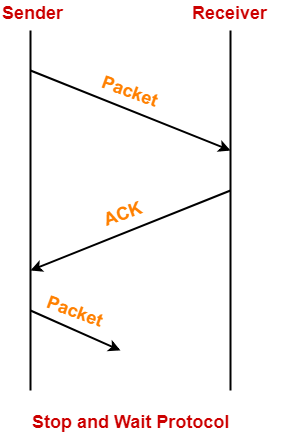The main problem faced by the Stop and Wait protocol is the occurrence of deadlock due to-

1. Loss of data packet
2. Loss of acknowledgement

## Stop and Wait ARQ-

 Stop and Wait ARQ is an improved and modified version of Stop and Wait protocol.

Stop and Wait ARQ assumes-

• The communication channel is noisy.
• Errors may get introduced in the data during the transmission.

## Working-

• Stop and wait ARQ works similar to stop and wait protocol.
• It provides a solution to all the limitations of stop and wait protocol.
• Stop and wait ARQ includes the following three extra elements.Thus, we can say-

 Stop and Wait ARQ= Stop and Wait Protocol + Time Out Timer + Sequence Numbers for Data Packets and Acknowledgements

## NOTE

For any sliding window protocol to work without any problem,

the following condition must be satisfied-

Available Sequence Numbers >= Sender Window Size + Receiver Window Size

Stop and wait ARQ is a one bit sliding window protocol where-

• Sender window size = 1
• Receiver window size = 1

Thus, in stop and wait ARQ,

Minimum number of sequence numbers required

= Sender Window Size + Receiver Window Size

= 1 + 1

= 2

Thus,

• Minimum number of sequence numbers required in Stop and Wait ARQ = 2.
• The two sequence numbers used are 0 and 1.

## 1. Problem of Lost Data Packet-

• After sending a data packet  to the receiver, sender starts the time out timer.
• If the data packet gets acknowledged before the timer expires, sender stops the time out timer.
• If the timer goes off before receiving the acknowledgement, sender retransmits the same data packet.
• After retransmission, sender resets the timer.
• This prevents the occurrence of deadlock.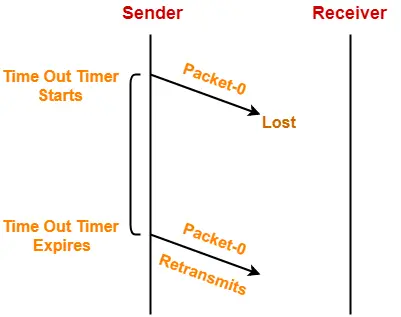## 2. Problem of Lost Acknowledgement-

• Sequence number on data packets help to solve the problem of delayed acknowledgement.
• Consider the acknowledgement sent by the receiver gets lost.
• Then, sender retransmits the same data packet after its timer goes off.
• This prevents the occurrence of deadlock.
• The sequence number on the data packet helps the receiver to identify the duplicate data packet.
• Receiver discards the duplicate packet and re-sends the same acknowledgement.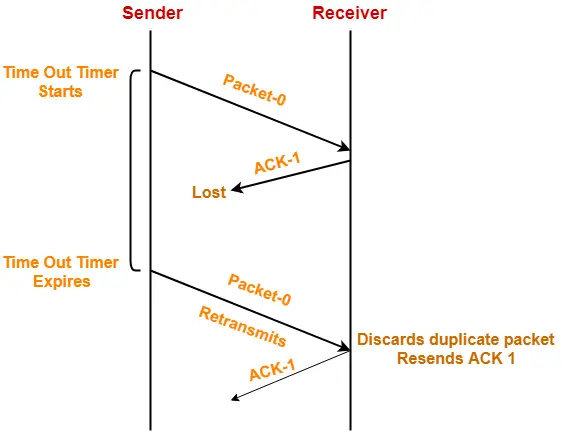## Role of Sequence Number on Data Packets

Consider the above example-

### Step-01:

• Sender sends a data packet with sequence number-0 to the receiver.

### Step-02:

• Receiver receives the data packet correctly.
• Receiver now expects data packet with sequence number-1.
• Receiver sends the acknowledgement ACK-1.

### Step-03:

• Acknowledgement ACK-1 sent by the receiver gets lost on the way.

### Step-04:

• Sender receives no acknowledgement and time out occurs.
• Sender retransmits the same data packet with sequence number-0.
• This will be a duplicate packet for the receiver.

### Step-05:

• Receiver receives the data packet and discovers it is the duplicate packet.
• It expects the data packet with sequence number-1 but receiving the data packet with sequence number-0.
• It discards the duplicate data packet and re-sends acknowledgement ACK-1.
• ACK-1 requests the sender to send a data packet with sequence number-1.
• This avoids the inconsistency of data.

### Conclusion-

• Had the sequence numbers not been allotted to the data packets, receiver would have accepted the duplicate data packet thinking of it as the new data packet.
• This is how sequence numbers allotted to the data packets prove to be useful for identifying the duplicate data packets and discarding them.

## 3. Problem of Delayed Acknowledgement-

• Sequence number on acknowledgements help to solve the problem of delayed acknowledgement.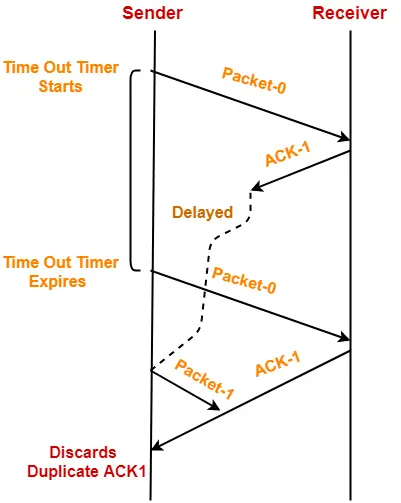## Role of Sequence Number on Acknowledgements

Consider the above example-

### Step-01:

• Sender sends a data packet with sequence number-0 to the receiver.

### Step-02:

• Receiver receives the data packet correctly.
• Receiver now expects data packet with sequence number-1.
• Receiver sends the acknowledgement ACK-1.

### Step-03:

• Acknowledgement ACK-1 sent by the receiver gets delayed in reaching the sender.

### Step-04:

• Sender receives no acknowledgement and time out occurs.
• Sender retransmits the same data packet with sequence number-0.
• This will be a duplicate packet for the receiver.

### Step-05:

• Receiver receives the data packet and discovers it is the duplicate packet.
• It expects the data packet with sequence number-1 but receiving the data packet with sequence number-0.
• It discards the duplicate data packet and re-sends acknowledgement ACK-1.
• ACK-1 requests the sender to send a data packet with sequence number-1.

### Step-06:

• Two acknowledgements ACK1 will reach the sender.
• When first acknowledgement ACK1 reaches the sender, sender sends the next data packet with sequence number 1.
• When second acknowledgement ACK1 reaches the sender, sender rejects the duplicate acknowledgement.
• This is because it has already sent the data packet with sequence number-1 and now sender expects the acknowledgement with sequence number 0 from the receiver.

### Conclusion-

• Had the sequence numbers not been allotted to the acknowledgements, sender would have accepted the duplicate acknowledgement thinking of it as the new acknowledgement for the latest data packet sent by it.
• This is how sequence numbers allotted to the acknowledgements prove to be useful for identifying duplicate acknowledgements and discarding them.

## 4. Problem of Damaged Packet-

• If receiver receives a corrupted data packet from the sender, it sends a negative acknowledgement (NAK) to the sender.
• NAK requests the sender to send the data packet again.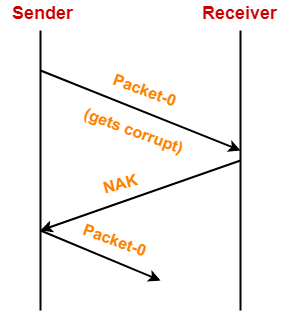## Stop and Wait Protocol Vs Stop and Wait ARQ-

The following comparison table states the differences between the two protocols-

 Stop and Wait Protocol Stop and Wait ARQ It assumes that the communication channel is perfect and noise free. It assumes that the communication channel is imperfect and noisy. Data packet sent by the sender can never get corrupt. Data packet sent by the sender may get corrupt. There is no concept of negative acknowledgements. A negative acknowledgement is sent by the receiver if the data packet is found to be corrupt. There is no concept of time out timer. Sender starts the time out timer after sending the data packet. There is no concept of sequence numbers. Data packets and acknowledgements are numbered using sequence numbers.

## Limitation of Stop and Wait ARQ-

 The major limitation of Stop and Wait ARQ is its very less efficiency.

To increase the efficiency, protocols like Go back N and Selective Repeat are used.

## Explanation-

In stop and wait ARQ,

• Sender window size is 1.
• This allows the sender to keep only one frame unacknowledged.
• So, sender sends one frame and then waits until the sent frame gets acknowledged.
• After receiving the acknowledgement from the receiver, sender sends the next frame.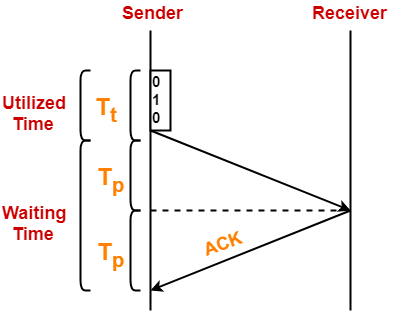Here,

• Sender uses Tt time for transmitting the packet over the link.
• Then, sender waits for 2 x Tp time.
• After 2 x Tp time, sender receives the acknowledgement for the sent frame from the receiver.
• Then, sender sends the next frame.
• This 2 x Tp waiting time is the actual cause of less efficiency.

## Efficiency Improvement-

• The efficiency of stop and wait ARQ can be improved by increasing the window size.
• This allows the sender to keep more than one unacknowledged frame in its window.
• Thus, sender can send frames in the waiting time too.

This gives rise to the concept of sliding window protocols.

To gain better understanding about Stop and Wait ARQ,

Watch this Video Lecture

Next Article- Practice Problems On Stop and Wait Protocol

Get more notes and other study material of Computer Networks.

Watch video lectures by visiting our YouTube channel LearnVidFun.